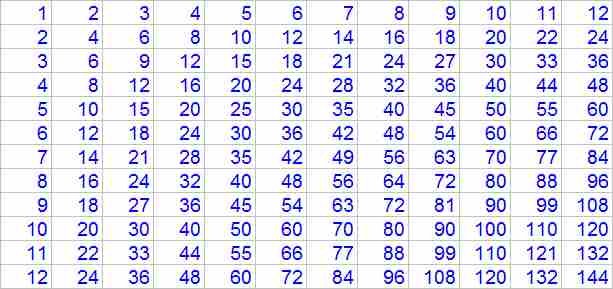Parents and Teachers: Support Ducksters by following us onor.

Kids Math

Multiplication Basics

What is multiplication?

Multiplication is when you take one number and add it together a number of times.

Example:

5 multiplied by 4 = 5 + 5 + 5 + 5 = 20

We took the number 5 and added it together 4 times. This is why multiplication is sometimes called "times".

More examples:
• 7 x 3 = 7 + 7 + 7 = 21
• 2 x 1 = 2
• 3 x 6 = 3 + 3 + 3 + 3 + 3 + 3 = 18
Signs for Multiplication

There are a few different signs that people use to indicate multiplication. The most common is the "x" sign, but sometimes people use a "*" sign or other symbols. Here are some ways to indicate 5 multiplied by 4.
• 5 x 4
• 5 * 4
• 5 times 4
Sometimes when people use variables in multiplication they will just put the variables next to each other to indicate multiplication. Here are a few examples:
• ab = a x b
• (a +1)(b + 1) = (a +1) x (b + 1)
Factors and Products

Sometimes when teachers talk about multiplication they will use the terms factors and products.

Factors are the numbers you are multiplying together. Products are the answers.

(factor) x (factor) = product

Multiplying by Zero and One

Zero and one are two special cases when multiplying.

When multiplying by 0, the answer is always 0.

Examples:
• 1 x 0 = 0
• 7676 x 0 = 0
• 0 x 12 = 0
• 0 x b = 0
When multiplying by 1, the answer is always the same as the number multiplied by 1.

Examples:
• 1 x 12 = 12
• 7654 x 1 = 7654
• 1 x 0 = 0
• 1 x b = b
Order Doesn't Matter

An important rule to remember with multiplication is that the order you multiply numbers doesn't matter. You can multiply them in any order you want and the answer will be the same. This can help sometimes when you get stuck on a problem. Just try it the other way.

Examples:
• 5 x 4 = 4 + 4 + 4 + 4 + 4 = 20
• 4 x 5 = 5 + 5 + 5 + 5 = 20

• 3 x 2 = 2 + 2 + 2 = 6
• 2 x 3 = 3 + 3 = 6

• 4 x 1 = 1 + 1 + 1 +1 = 4
• 1 x 4 = 4 = 4
Multiplication Table

Once you learn the basics of multiplication, you will want to learn the multiplication table, also called the times table. This table includes all of the possible multiplications between the numbers 1 to 12. That is all the way from 1 x 1 to 12 x 12.

It may sound like a lot of useless work to memorize this table, but it will help you a LOT later in school. You will be able to solve harder problems faster and easier if you know these numbers by heart.

Here is the table:Click on the table to get a larger version you can print out.

 Multiplication Intro to Multiplication Long Multiplication Multiplication Tips and Tricks Division Intro to Division Long Division Division Tips and Tricks Fractions Intro to Fractions Equivalent Fractions Simplifying and Reducing Fractions Adding and Subtracting Fractions Multiplying and Dividing Fractions Decimals Decimals Place Value Adding and Subtracting Decimals Multiplying and Dividing Decimals Statistics Mean, Median, Mode, and Range Picture Graphs Algebra Order of Operations Exponents Ratios Ratios, Fractions, and Percentages Geometry Polygons Quadrilaterals Triangles Pythagorean Theorem Circle Perimeter Surface Area Misc Basic Laws of Math Prime Numbers Roman Numerals Binary Numbers

Back to Kids Math

Back to Kids StudyFollow us onor